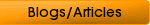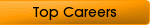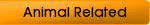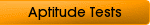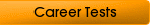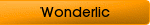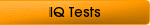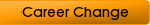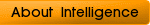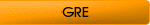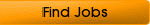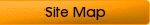## Elements of Numerical Aptitude Tests

What would it take to pass numerical aptitude tests? Why are they necessary anyway? In a world where technology never ceases to improve, you should brace yourself and prepare well, in a mathematical sense, at least. Most employers nowadays require that you pass a numerical aptitude test before you can even move to the next step of the job hiring process.

Maybe you just do not remember it, but you have surely taken some numerical aptitude test at some point in your life, especially in grade school and high school. Today, this is pretty much a standard when you apply for a job, especially in careers that involve some form of calculations or mathematics. Numerical aptitude tests are used to measure your ability to execute tasks that involve statistics, numbers, and other mathematical functions such as addition, subtraction, multiplication, and others. These tests also measure your logical thinking through determining if you can identify what mathematical formulas to use if you were given a problem. In some cases, the tests will also require you to interpret numbers in the form of graphs and tables.

Just like many aptitude tests, numerical aptitude tests are timed. The test facilitators ensure that everyone complies with this time requirement because extending the time defeats the purpose of having the test. In some facilities, the tests are already done through computers. Normally, the people taking the test are given only 30-40 minutes to complete the entire exam.

Given this short time frame, you might get worried that you won’t finish the test. You should not be bothered by this since what really sums up is the number of correct answers that you got, regardless if you finished the test or not. This is especially so if the kind of job you are applying for will require daily crunching of numbers or figures. In a typical career setting, employers who let you undergo numerical aptitude tests want to see how you will eventually perform in the job through the results of the tests.

### Types of the Test

Today, there rae hundreds of numerical aptitude tests available to those who want to know their mathematical prowess. The difficulty levels also vary since these tests are used in different settings, for different purposes, and for different targets. However, the most common types of tests are the following:

Logical

These types of numerical aptitude tests measure your ability to analyze problems. What these tests measure is your reasoning but not your ability to calculate. An example of this test is choosing the missing number in a series of numbers.

Computation

This type of test will require you to use the basic mathematical functions such as addition, multiplication, and so on. These tests also involve other mathematical principles such as fractions, decimals, and ratios. The answers required for the questions in these tests are accurate answers.

Interpretation

In some tests, you will be presented graphs such as bar or pie charts and you will choose the closes analysis based on the graph. This type of tests is usually administered in managerial positions or for jobs that require analytical and statistical skills.

Estimation

Some test questions are designed to be difficult and the choices do not have the correct answers. What you are required to do in this type of tests is to simply estimate the range or the closest answer to the question.

### How to Pass the Test

Below are tips that you need to do or remember to make sure you pass that test and move on to the next level of the hiring process.

• The basic mathematical skills you should possess are addition, subtraction, division, and multiplication.

• Some mathematical concepts that will pop up in these tests are percentages, decimals, fractions, and integers.

• Get a math book exercise and answer the questions without a calculator. This should get you used to the process of calculating and thinking fast. Use a scratch paper and time yourself.

• Make it a habit to answer puzzles in newspapers. Do crossword puzzles as well since this activity enhances reasoning and logical thinking.

• Calculate all the time. For example, calculate gas prices in your head. Calculate grocery totals when buying things. Try to think in terms of numbers and create mathematical problems to solve.

• Read and understand stock market graphs in the newspaper.

Sure enough, you want to pass the test. You might say you are not so good at math and it is not likely that you will pass numerical aptitude tests. That is not entirely true. Anybody who has a basic grasp of mathematics and algebra should pass this test. All it takes is consistent practice.

Home
Numerical Test
Algebra Test
Math Aptitude Test